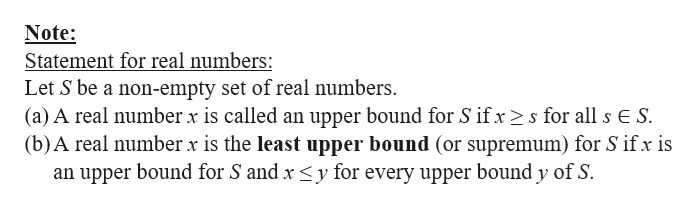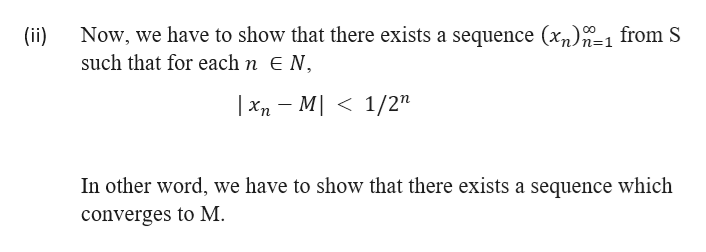# Let S ⊆ R, and recall M = supS is the least upper bound of S. Suppose that S is bounded, so supS ∈ R. (If S was not bounded, then we set supS = ∞). Show that for every ε > 0, there exists x ∈ S such that |x−M| < ε and that there exists a sequence (xn) ∞n=1 from S such that for each n ∈ N,|xn − M| < 1/2n.  Define M to be the supremum of S. R and N are the real numbers and natural numbers, respectively.

Question
48 views
1. Let S ⊆ R, and recall M = supS is the least upper bound of S. Suppose that S is bounded, so supS ∈ R. (If S was not bounded, then we set supS = ∞). Show that for every ε > 0, there exists x ∈ S such that |x−M| < ε and that there exists a sequence (xn) n=1 from S such that for each n ∈ N,

|x− M| < 1/2n

Define M to be the supremum of S. R and N are the real numbers and natural numbers, respectively.

check_circle

Step 1

Let S ⊆ R and M = sup S which is the least upper bound of S. We are given that S is bounded, so sup S ∈ R.

(i)   We have to show that for every ε > 0, there exists x ∈ S such that  |x − M| < ε .help_outlineImage TranscriptioncloseNote: Statement for real numbers: Let S be a non-empty set of real numbers (a) A real number x is called an upper bound for S if xs for all s E S (b)A real number x is the least upper bound (or supremum) for S if x is an upper bound for S and x
Step 2

Proof of (i)

If M is a least upper bound then for every ε > 0 there exist x ∈ S such that  M – ε < x, if not, M – ε will be the least upper bound of the set S by its definition. Also, x ≤ M by the definition of least upper bound.

So, it is trivial that x ≤ M+ ε.

Hence,

M – ε < x ≤ M+ ε

or      |x − M| < ε

Step 3

Again,

...help_outlineImage TranscriptioncloseNow, we have to show that there exists a sequence (x1)7-1 from S (ii) such that for each n E N, |xn- MI1/2n In other word, we have to show that there exists a sequence which converges to M fullscreen

### Want to see the full answer?

See Solution

#### Want to see this answer and more?

Solutions are written by subject experts who are available 24/7. Questions are typically answered within 1 hour.*

See Solution
*Response times may vary by subject and question.
Tagged in

### Math